# Colleges with the highest SAT scores in New Jersey

Top 10 colleges in New Jersey with the highest SAT scores
Looking for the colleges with the highest SAT scores in New Jersey? Well you're in luck! We've compiled a national college database and have created a list of the top 10 universities with the highest SAT scores in New Jersey. These are the schools whose applicants had the highest average SAT scores in New Jersey. And, since these tests are meant to determine academic prowess, they are arguably the schools with the most academically proficient students. You could even say these are the best colleges in New Jersey. We also include each college's ACT scores and acceptance rate so that you can see where you would have the easiest or hardest time getting in. Read on to find out more.

## Princeton University SAT scores

The average SAT score for Princeton University is 1505.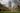The average SAT score of 1505 breaks down into:

• SAT math: 765

The average ACT score for Princeton University is 34 and their acceptance rate is 5.5%.

## Stevens Institute of Technology SAT scores

The average SAT score for Stevens Institute of Technology is 1405.
The average SAT score of 1405 breaks down into:

• SAT math: 730

The average ACT score for Stevens Institute of Technology is 32 and their acceptance rate is 41.4%.

## Rutgers University SAT scores

The average SAT score for Rutgers University is 1300.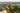The average SAT score of 1300 breaks down into:

• SAT math: 665

The average ACT score for Rutgers University is 28 and their acceptance rate is 60.1%.

## New Jersey Institute of Technology SAT scores

The average SAT score for New Jersey Institute of Technology is 1290.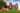The average SAT score of 1290 breaks down into:

• SAT math: 660

The average ACT score for New Jersey Institute of Technology is 27 and their acceptance rate is 63.7%.

## The College of New Jersey SAT scores

The average SAT score for The College of New Jersey is 1260.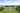The average SAT score of 1260 breaks down into:

• SAT math: 635

The average ACT score for The College of New Jersey is 28 and their acceptance rate is 49.3%.

## Seton Hall University SAT scores

The average SAT score for Seton Hall University is 1230.The average SAT score of 1230 breaks down into:

• SAT math: 615

The average ACT score for Seton Hall University is 26 and their acceptance rate is 70.4%.

## Drew University SAT scores

The average SAT score for Drew University is 1212.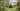The average SAT score of 1212 breaks down into:

• SAT math: 600

The average ACT score for Drew University is 27 and their acceptance rate is 69.2%.

## Ramapo College of New Jersey SAT scores

The average SAT score for Ramapo College of New Jersey is 1150.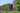The average SAT score of 1150 breaks down into:

• SAT math: 570

The average ACT score for Ramapo College of New Jersey is 24 and their acceptance rate is 57.4%.

## Monmouth University SAT scores

The average SAT score for Monmouth University is 1130.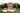The average SAT score of 1130 breaks down into:

• SAT math: 560

The average ACT score for Monmouth University is 23 and their acceptance rate is 77.3%.

## Richard Stockton College SAT scores

The average SAT score for Richard Stockton College is 1102.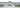The average SAT score of 1102 breaks down into:

• SAT math: 550